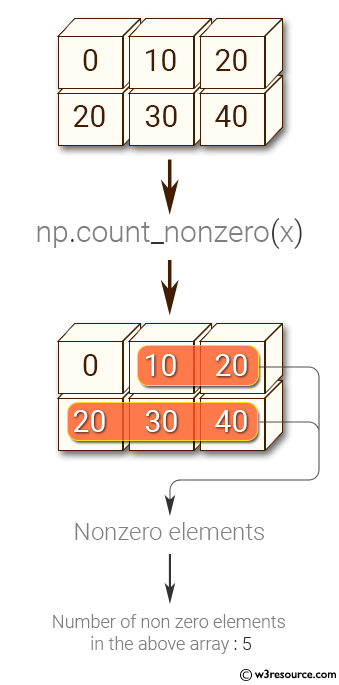﻿ NumPy: Get the number of nonzero elements in an array - w3resource# NumPy: Get the number of nonzero elements in an array

## NumPy: Array Object Exercise-63 with Solution

Write a NumPy program to get the number of nonzero elements in an array.

Pictorial Presentation:Sample Solution:-

Python Code:

``````import numpy as np
x = np.array([[0, 10, 20], [20, 30, 40]])
print("Original array:")
print(x)
print("Number of non zero elements in the above array:")
print(np.count_nonzero(x))
```
```

Sample Output:

```Original array:
[[ 0 10 20]
[20 30 40]]
Number of non zero elements in the above array:
5
```

Python Code Editor:

Have another way to solve this solution? Contribute your code (and comments) through Disqus.

What is the difficulty level of this exercise?

Test your Python skills with w3resource's quiz

﻿

## Python: Tips of the Day

Set comprehension:

```>>> m = {x ** 2 for x in range(5)}
>>> m
{0, 1, 4, 9, 16}
```